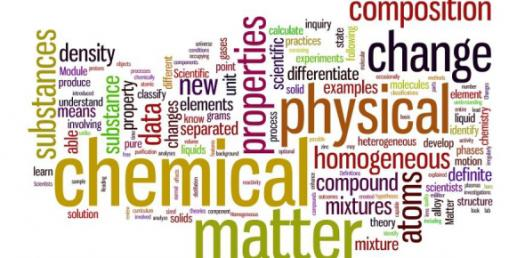# Quiz: Can You Solve The Chemistry Exam Test?

27 Questions | Total Attempts: 1189Settings.

• 1.
Helium atoms do not combine to form He2 molecules, yet He atoms do attract one another weakly through?
• A.

Dispersion forces

• B.

Ion-dipole forces

• C.

Dipole-dipole forces

• D.

Hydrogen bonding

• 2.
Which one of the following substances should exhibit hydrogen bonding in the liquid state?
• A.

H2

• B.

He

• C.

H2S

• D.

H2O

• 3.
In which one of the following substances will the individual molecules experience both London forces and dipole-dipole forces?
• A.

BCL3

• B.

HCl

• C.

Br2

• D.

CO2

• 4.
Which of the following substances should have the highest boiling point?
• A.

CH4

• B.

Cl 2

• C.

N2

• D.

CH3OH

• 5.
Which of the following liquids should have the highest viscosity at the same temperature?
• A.

CH3OCH3

• B.

CH3Cl2

• C.

HOCH2CH2OH

• D.

CH3Br

• 6.
Which of the following substances would be expected to have the lowest vapor pressure at room temperature?
• A.

Ethanol bp=78 degrees celsius

• B.

Methanol bp=65 degrees celsius

• C.

Water bp=100 degrees celsius

• D.

Acetone bp=56 degrees celsius

• 7.
Which one of the following substances should exhibit hydrogen bonding in the liquid state?
• A.

CH3COOH

• B.

CH4

• C.

PH3

• D.

He

• 8.
The lattice points in a crystal lattice may be occupied by?
• A.

Ions

• B.

Neutral atoms

• C.

Neutral molecules

• D.

Any of the above

• 9.
Platinum has a face-centered cubic crystal structure with a unit cell length of 392pm. What is the radius of the platinum atom?
• A.

69pm

• B.

98pm

• C.

277pm

• D.

139pm

• 10.
The vapor pressure of a liquid in a closed container depends upon?
• A.

The amound of liquid

• B.

The surface area of the liquid

• C.

The volume of the container

• D.

The temperature

• 11.
Which one of the following would be immiscible with water?
• A.

S=C=S

• B.

C2H5OH

• C.

CH3OH

• D.

NH3

• 12.
Calculate the amount of heat needed to melt 2.0 kg of iron at its melting point (1809K) given that? delta Hfus=133.80kJ/mol.
• A.

494 kJ

• B.

27.6 kJ

• C.

25,000 kJ

• D.

27,600 kJ

• 13.
Which of the following compounds will be soluble in CCl4?
• A.

NaCl

• B.

H2O

• C.

C8H18

• D.

NaOH

• 14.
Which of the following usually represents the strongest type of intermolecular force?
• A.

Ion-dipole

• B.

H-bonds

• C.

Dispersion

• D.

Dipole-dipole

• 15.
What is the percent CdSO4 by mass in a 1.0 molal aqueous solution (MW of CdSO4 =208.46 g/mol)?
• A.

0.10%

• B.

17.2%

• C.

20.8%

• D.

24.4%

• 16.
Calculate the percent by mass of potassium nitrate in a solution made from 45.0g KNO3 and 295 mL of water. Density of water is 0.997 g/mL.
• A.

15.2%

• B.

1.51%

• C.

7.57%

• D.

13.3%

• 17.
Calculate the molality of a solution containing 14.3 g of NaCl in 42.2 g of water.
• A.

2.45 x 10-4 m

• B.

5.80 x 10-4 m

• C.

2.45 x 10-1 m

• D.

5.79 m

• 18.
Which of the following solution would have the highest boiling point?
• A.

0.1 m glucose in water

• B.

0.1m glycerol in water

• C.

0.1m NaCl in water

• D.

Pure water

• 19.
What is the approximate Na+ ion concentration in a 0.75 M Na2CO3 solution? Na2CO3 is a salt which will fully dissociate
• A.

1.50M

• B.

0.375 M

• C.

0.75M

• D.

1.25M

• 20.
Calculate the mole fraction of CH3CH2OH ethanol(molar mass=46.0 g/mol), in a solution that contains 46g of ethanol and 64g of CH3OH.
• A.

0.33

• B.

0.42

• C.

0.50

• D.

0.67

• 21.
As a non-volatile solute is added to a pure solvent, which of the following statements is true?
• A.

As the solute is added, the boiling point will decrease and the freezing point will increase

• B.

As the solute is added, the boiling point will increase and the freezing point will decrease

• C.

As the solute is added, both the boiling point and the freezing point will decrease

• D.

As the solute is added, both the boiling point and the freezing point will increase

• 22.
Calculate the amount of heat theat must be absorbed by 10.0 g of ice at -20 degrees C to convert it to liquid water at 60.0 degrees C. Given: Specific heat (ice)=2.1 J/g degrees C Specific heat (water) =4.18 J/g degrees C delta Hfus=6.0 kJ/mol
• A.

6.112 kJ or 6,112 J

• B.

6.26 kJ or 6,261 J

• C.

5.26 kJ or 5,260 J

• D.

4.36 kJ or 4,360 J

• 23.
The vapor pressure of ethanol is 400 mmHg at 63.5 degrees C. Its molar heat of vaporization is 39.3 kJ/mol. What is the vapor pressure of ethanol in mmHg at 34.9 degrees C? The gas constant R, equals 8.314 J/mol*K
• A.

P2=105.5 mmHg

• B.

P2=103.5 mmHg

• C.

P2=108.5 mmHg

• D.

P2=117.2 mmHg

• 24.
Acetic acid has a heat of fusion of 10.8 kJ/mol and a heat of vaporization of 24.3 kJ/mol. What is the expected value of the heat of sublimation of acetic acid?
• A.

22.1 kJ/mol

• B.

32.1 kJ/mol

• C.

35.1 kJ/mol

• D.

40.1 kJ/mol

• 25.
What is the molarity of a solution that is 22.3 % by mass sulfuric acid (H2SO4,MW 98.08 g/mol) and that has a density of 1.136 g/mL?
• A.

1.23 mol/L

• B.

1.55 mol/L

• C.

2.58 mol/L

• D.

2.254 mol/L

Related TopicsBack to top